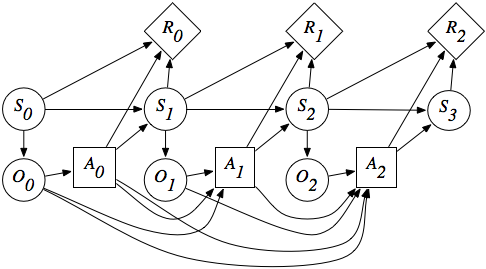### 9.5.6 Partially Observable Decision Processes

A partially observable Markov decision process (POMDP) is a combination of an MDP and a hidden Markov model. Instead of assuming that the state is observable, we assume that there are some partial and/or noisy observations of the state that the agent gets to observe before it has to act.

A POMDP consists of the following:

• S, a set of states of the world;
• A, a set of actions;
• O, a set of possible observations;
• P(S0), which gives the probability distribution of the starting state;
• P(S'|S,A), which specifies the dynamics - the probability of getting to state S' by doing action A from state S;
• R(S,A,S'), which gives the expected reward of starting in state S, doing action A, and transitioning to state S'; and
• P(O|S), which gives the probability of observing O given the state is S.

A finite part of a POMDP can be depicted using the decision diagram as in Figure 9.19.Figure 9.19: A POMDP as a dynamic decision network

There are three main ways to approach the problem of computing the optimal policy for a POMDP:

• Solve the associated dynamic decision network using variable elimination for decision networks [Figure 9.11, extended to include discounted rewards]. The policy created is a function of the history of the agent. The problem with this approach is that the history is unbounded, and the number of possible histories is exponential in the planning horizon.
• Make the policy a function of the belief state - a probability distribution over the states. Maintaining the belief state is the problem of filtering. The problem with this approach is that, with n states, the set of belief states is an (n-1)-dimensional real space. However, because the value of a sequence of actions only depends on the states, the expected value is a linear function of the values of the states. Because plans can be conditional on observations, and we only consider optimal actions for any belief state, the optimal policy for any finite look-ahead, is piecewise linear and convex.
• Search over the space of controllers for the best controller. Thus, the agent searches over what to remember and what to do based on its belief state and observations. Note that the first two proposals are instances of this approach: the agent remembers all of its history or the agent has a belief state that is a probability distribution over possible states. In general, the agent may want to remember some parts of its history but have probabilities over some other features. Because it is unconstrained over what to remember, the search space is enormous.